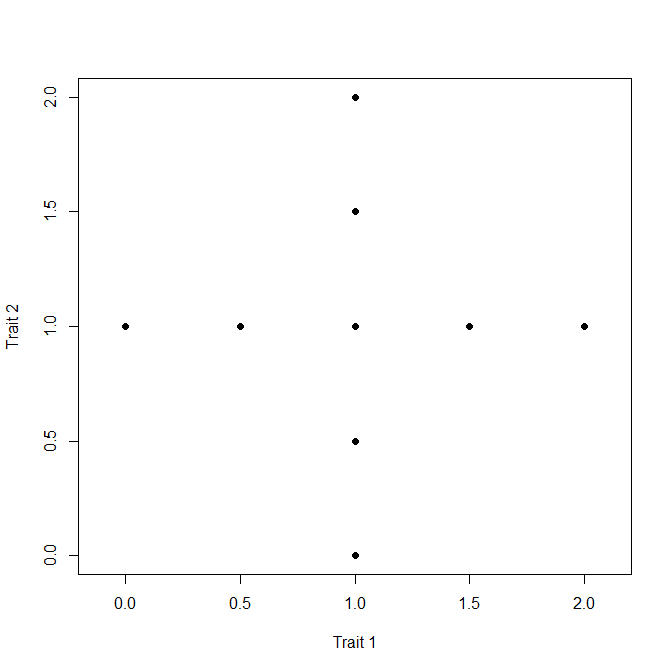# 4. Check Numerical Correctness of Indices

Most research results nowadays rely heavily on results generated by computational software. Yet, this software, while occupying an increasingly central place in science, is often poorly tested. Users are never presented any arguments about why they should trust the code provided to them.

We want to contribute to change this trend and in this vignette, we show that indices computed by fundiversity behave as theoretically expected, and as presented in Villéger, Mason, and Mouillot (2008). We also internally compared fundiversity results with ones obtained by other packages. You can see the equivalence between functions in the benchmark vignette that compares fundiversity and alternative packages.

library(fundiversity)

For this, we reproduce the Figure 2 (including all of their facets) of their article. If you re-use this figure, please cite the original article.

We start by reproducing Figure 2 a, to check that the values computed by fundiversity are correct. These are the data found in Figure 2 of the article:

data_a <- matrix(byrow = TRUE, ncol = 2,
c(
0.0, 1.0,
0.5, 1.0,
1.0, 1.0,
1.5, 1.0,
2.0, 1.0,
1.0, 0.0,
1.0, 0.5,
1.0, 1.5,
1.0, 2.0
)
)
rownames(data_a) <- paste0("species", seq_len(nrow(data_a)))
print(data_a)
#>          [,1] [,2]
#> species1  0.0  1.0
#> species2  0.5  1.0
#> species3  1.0  1.0
#> species4  1.5  1.0
#> species5  2.0  1.0
#> species6  1.0  0.0
#> species7  1.0  0.5
#> species8  1.0  1.5
#> species9  1.0  2.0
plot(data_a, pch = 19, asp = 1, xlab = "Trait 1", ylab = "Trait 2")We then compute the functional diversity indices.

fric_a <- fd_fric(data_a)[["FRic"]]
feve_a <- fd_feve(data_a)[["FEve"]]
fdiv_a <- fd_fdiv(data_a)[["FDiv"]]
fdis_a <- fd_fdis(data_a)[["FDis"]]
raoq_a <- fd_raoq(data_a)[["Q"]]

As expected, we found an FRic value of 2 (should be 2), an FEve value of 1 (should be 1), and a FDiv value of 0.692 (should be 0.692).

# Changes of abundance

If we change the abundance, but not the coordinates (i.e., not the species characteristics), we expect changes in FEve and FDiv but not FRic.

More precisely, equal increases in abundance for the species at the vertices of the convex hull don’t influence FEve, but lead to a higher FDiv. These correspond to the data found in panel c of the Figure 2.

l <- 2 # common species
s <- 1 # rare species

wc <- matrix(c(l, s, l, s, l, l, s, s, l), nrow = 1)
colnames(wc) <- rownames(data_a)
rownames(wc) <- "site"

We compute again the functional diversity indices:

fric_c <- fd_fric(data_a)[["FRic"]]
feve_c <- fd_feve(data_a, wc)[["FEve"]]
fdiv_c <- fd_fdiv(data_a, wc)[["FDiv"]]
fdis_c <- fd_fdis(data_a, wc)[["FDis"]]
raoq_c <- fd_raoq(data_a, wc)[["Q"]]

As expected, we found an FRic value of 2 (should be 2), an FEve value of 1 (should be 1), and a FDiv value of 0.714 (should be 0.714).

Conversely, changes of abundances on a single trait axis don’t impact FDiv but reduce FEve (this correspond to the panel b of Figure 2):

l <- 2 # common species
s <- 1 # rare species

wb <- matrix(c(l, l, l, l, l, s, s, s, s), nrow = 1)
colnames(wb) <- rownames(data_a)
rownames(wb) <- "site"

We can compute weighted functional diversity indices:

fric_b <- fd_fric(data_a)[["FRic"]]
feve_b <- fd_feve(data_a, wb)[["FEve"]]
fdiv_b <- fd_fdiv(data_a, wb)[["FDiv"]]
fdis_b <- fd_fdis(data_a, wb)[["FDis"]]
raoq_b <- fd_raoq(data_a, wb)[["Q"]]

As expected, we found an FRic value of 2 (should be 2), an FEve value of 0.8571429 (should be 0.778), and a FDiv value of 0.659 (should be 0.692).

# Changes of coordinates (= traits)

We now change the species characteristics (i.e., the coordinates) instead of the abundances. The changes of coordinates without changing abundances can affect all diversity indices. If the coordinates are only changed for species that are not on the convex hull would only affect FEve and FDiv.

For example, we can change the coordinates of species to be at the same distance of the centroid of the species, so that it doesn’t affect the value of FDiv, but put them further away from points on the convex hull. This would decrease FEve because species would be spaced less evenly in the space (it corresponds to panel d of Figure 2):

shift <- 1/(2*sqrt(2))

data_d <- matrix(c(
1-shift, 1-shift,
1-shift, 1+shift,
1+shift, 1-shift,
1+shift, 1+shift,
1.00 , 0.00 ,
2.00 , 1.00 ,
1.00 , 2.00 ,
0.00 , 1.00 ,
1.00 , 1.00),
byrow = TRUE,
ncol = 2
)
fric_d <- fd_fric(data_d)[["FRic"]]
feve_d <- fd_feve(data_d)[["FEve"]]
fdiv_d <- fd_fdiv(data_d)[["FDiv"]]
fdis_d <- fd_fdis(data_d)[["FDis"]]
raoq_d <- fd_raoq(data_d)[["Q"]]

As expected, we found an FRic value of 2 (should be 2), an FEve value of 0.891 (should be 0.891), and a FDiv value of 0.692 (should be 0.692).

We can also change the coordinates of species (their traits) to only affect FDiv and not FEve by, instead, moving species on the convex hull while keeping their distance to other points equal. This corresponds to the panel e Figure 2 of the paper:

data_e <- matrix(c(
0.0, 1.0,
0.5, 0.5,
1.0, 1.0,
1.5, 0.5,
2.0, 1.0,
1.0, 0.0,
0.5, 1.5,
1.5, 1.5,
1.0, 2.0),
byrow = TRUE,
ncol = 2
)

We can then compute functional diversity indices:

fric_e <- fd_fric(data_e)[["FRic"]]
feve_e <- fd_feve(data_e)[["FEve"]]
fdiv_e <- fd_fdiv(data_e)[["FDiv"]]
fdis_e <- fd_fdis(data_e)[["FDis"]]
raoq_e <- fd_raoq(data_e)[["Q"]]

As expected, we found an FRic value of 2 (should be 2), an FEve value of 1 (should be 1), and a FDiv value of 0.78 (should be 0.78).

Villéger, Sébastien, Norman W. H. Mason, and David Mouillot. 2008. “New Multidimensional Functional Diversity Indices for a Multifaceted Framework in Functional Ecology.” Ecology 89 (8): 2290–2301. https://doi.org/10.1890/07-1206.1.